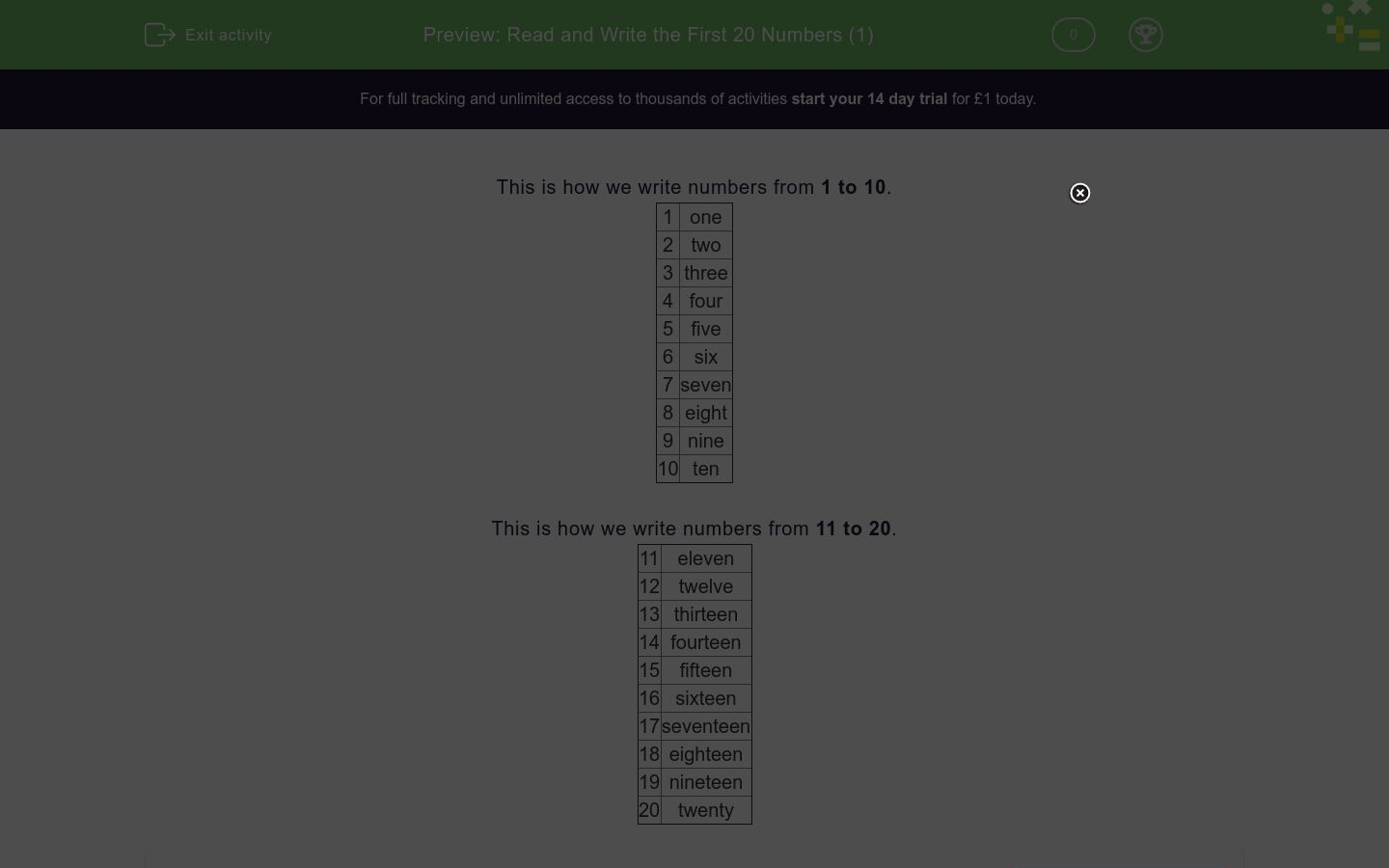# Read and Write the First 20 Numbers (1)

In this worksheet, children must read number words and select the correct number written in numerals.Key stage:  KS 1

Curriculum topic:  Number: Number and Place Value

Curriculum subtopic:  Read and Write Number Words to 20

Difficulty level:### QUESTION 1 of 10

This is how we write numbers from 1 to 10.

one

two

3    three

4    four

5    five

6    six

seven

8    eight

9    nine

10  ten

This is how we write numbers from 11 to 20.

11   eleven

12   twelve

13   thirteen

14   fourteen

15   fifteen

16   sixteen

17   seventeen

18   eighteen

19   nineteen

20   twenty

Choose the correct digits for this number:

six

2

16

6

9

Choose the correct digits for this number:

two

2

16

6

9

Choose the correct digits for this number:

sixteen

2

16

6

9

Choose the correct digits for this number:

nine

2

16

6

9

Choose the correct digits for this number:

four

4

15

12

7

Choose the correct digits for this number:

seven

4

15

12

7

Choose the correct digits for this number:

twelve

4

15

12

7

Choose the correct digits for this number:

fifteen

4

15

5

50

Choose the correct digits for this number:

one

4

10

1

7

Choose the correct digits for this number:

twenty

2

10

12

20

• Question 1

Choose the correct digits for this number:

six

6
EDDIE SAYS
six is 6
• Question 2

Choose the correct digits for this number:

two

2
EDDIE SAYS
two is 2
• Question 3

Choose the correct digits for this number:

sixteen

16
EDDIE SAYS
sixteen is 16
• Question 4

Choose the correct digits for this number:

nine

9
EDDIE SAYS
nine is 9
• Question 5

Choose the correct digits for this number:

four

4
EDDIE SAYS
four is 4
• Question 6

Choose the correct digits for this number:

seven

7
EDDIE SAYS
seven is 7
• Question 7

Choose the correct digits for this number:

twelve

12
EDDIE SAYS
twelve is 12
• Question 8

Choose the correct digits for this number:

fifteen

15
EDDIE SAYS
fifteen is 15
• Question 9

Choose the correct digits for this number:

one

1
EDDIE SAYS
one is 1
• Question 10

Choose the correct digits for this number:

twenty